Next: Isotharmal shock Up: Shock Wave Previous: Shock Wave   Contents

## Rankine-Hugoniot Relation

Passing through a shock front moving with a speed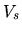, the physical variables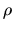,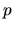, and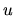change abruptly. Since the basic equations of hydrodynamics is unchanged after chosing a system moving, the continuity equation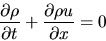(A.26)

gives an equation for a steady state as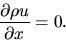(A.27)

Considering a region containing the shock front at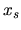which extends from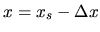to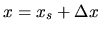and integrating the above equation, we get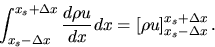(A.28)

Thus, we obtain the jump condition conserning the mass coservation as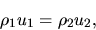(A.29)

where the quatities with suffix 1 are for preshock and those with suffix 2 are for postshock.

Equation of motion for steady state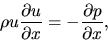(A.30)

gives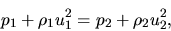(A.31)

where we used equation(A.29).

SubsectionsNext: Isotharmal shock Up: Shock Wave Previous: Shock Wave   Contents
Kohji Tomisaka 2007-07-08